Sound Waves and Music - Lesson 5 - Physics of Musical Instruments

# Closed-End Air Columns

In the previous part of Lesson 5, the formation of a standing wave patterns in an open-end instrument was discussed. The mathematics of the harmonic frequencies associated with such standing wave patterns were developed. This part of Lesson 5 will use similar principles to develop the standing wave patterns and associated mathematics for closed-end air column. An instrument consisting of a closed-end column typically contains a metal tube in which one of the ends is covered and not open to the surrounding air. Some pipe organs and the air column within the bottle of a pop-bottle orchestra are examples of closed-end instruments. Some instruments that operate as open-end air columns can be transformed into closed-end air columns by covering the end opposite the mouthpiece with a mute. As we will see the presence of the closed end on such an air column will affect the actual frequencies that the instrument can produce.

### Standing Wave Patterns for Harmonics

As has already been mentioned, a musical instrument has a set of natural frequencies at which it vibrates at when a disturbance is introduced into it. These natural frequencies are known as the harmonics of the instrument. Each harmonic is associated with a standing wave pattern. In Lesson 4 of Unit 10, a standing wave pattern was defined as a vibrational pattern created within a medium when the vibrational frequency of the source causes reflected waves from one end of the medium to interfere with incident waves from the source in such a manner that specific points along the medium appear to be standing still. In the case of stringed instruments (discussed earlier), standing wave patterns were drawn to depict the amount of movement of the string at various locations along its length. Such patterns show nodes - points of no displacement or movement - at the two fixed ends of the string. In the case of air columns, a closed end in a column of air is analogous to the fixed end on a vibrating string. That is, at the closed end of an air column, air is not free to undergo movement and thus is forced into assuming the nodal positions of the standing wave pattern. Air at the closed end of an air column is still. Conversely, air is free to undergo its back-and-forth longitudinal vibration at the open end of an air column. And as such, the standing wave patterns will depict vibrational antinodes at the open ends of air columns.

So the basis for drawing the standing wave patterns for air columns is that vibrational antinodes will be present at any open end and vibrational nodes will be present at any closed end. If this principle is applied to closed-end air columns, then the pattern for the fundamental frequency (the lowest frequency and longest wavelength pattern) will have a node at the closed end and an antinode at the open end. For this reason, the standing wave pattern for the fundamental frequency (or first harmonic) for a closed-end air column looks like the diagram below.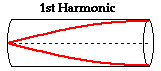The distance between adjacent antinodes on a standing wave pattern is equivalent to one-half of a wavelength. Since nodes always lie midway in between the antinodes, the distance between an antinode and a node must be equivalent to one-fourth of a wavelength. A careful analysis of the diagram above shows that a node and an adjacent antinode are positioned at the two ends of the air column. Thus, the length of the air column is equal to one-fourth of the wavelength for the first harmonic.

The fundamental frequency is the lowest possible frequency that any instrument can play; it is sometimes referred to as the first harmonic of the instrument. The second harmonic of any instrument always has a frequency that is twice the frequency of the first harmonic. The fourth harmonic of any instrument always has a frequency that is four times the frequency of the first harmonic. As we will see, a strange pattern results for a closed-end air column. Just as for all the instruments, the next harmonic for a closed-end air column is the harmonic that has one more node. And just as for all the instruments, the addition of an extra node also means that an extra antinode must also be added to the pattern. This would result in a total of two vibrational antinodes and one vibrational node. This pattern is shown in the diagram below. Observe in the pattern that there is three-fourths of a full wave in the length of the air column. That is three times the number of waves in the first harmonic. Since, the frequency of this harmonic is three times the frequency of the first harmonic, this is called the third harmonic.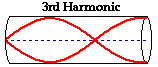But what happened to the second harmonic? Unlike the other instrument types, there is no second harmonic for a closed-end air column. The next frequency above the fundamental frequency is the third harmonic (three times the frequency of the fundamental). In fact, a closed-end instrument does not possess any even-numbered harmonics. Only odd-numbered harmonics are produced, where the frequency of each harmonic is some odd-numbered multiple of the frequency of the first harmonic.

The next highest frequency above the third harmonic is the fifth harmonic. It is the standing wave pattern with the next smallest wavelength. The standing wave pattern for the fifth harmonic of a closed-end air column is produced by adding another node to the pattern. This would result in a total of three anti-nodes and three nodes. This pattern is shown in the diagram below. Observe in the pattern that there are one and one-fourth waves present in the length of the air column. That is five times the number of waves in the first harmonic. For this reason, the frequency of the fifth harmonic is five times the frequency of the first harmonic.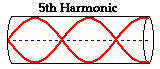The process of adding another node and antinode to each consecutive harmonic in order to determine the pattern and the resulting length-wavelength relationship could be continued. If doing so, it is important to keep vibrational antinodes on the open ends and vibrational nodes on the closed end of the air column and to maintain an alternating pattern of nodes and antinodes. When finished, the results should be consistent with the information in the table below.

### Length-Wavelength Relatiionships

The relationships between the standing wave pattern for a given harmonic and the length-wavelength relationships for closed-end air columns are summarized below.

 Harmonic # # of Waves in Column # of Nodes # of Antinodes Length- Wavelength Relationship1 1/4 1 1 λ = (4/1)*L3 3/4 2 2 λ = (4/3)*L5 5/4 3 3 λ = (4/5)*L7 7/4 4 4 λ = (4/7)*L9 9/4 5 5 λ = (4/9)*L

(The symbol λ represents the wavelength.)

### Problem-Solving Scheme

Now the aim of the above discussion is to internalize the mathematical relationships for closed-end air columns in order to perform calculations predicting the length of air column required to produce a given natural frequency. And conversely, calculations can be performed to predict the natural frequencies produced by a known length of air column. Each of these calculations requires knowledge of the speed of a wave in air (which is approximately 340 m/s at room temperatures). The graphic below depicts the relationships between the key variables in such calculations. These relationships will be used to assist in the solution to problems involving standing waves in musical instruments.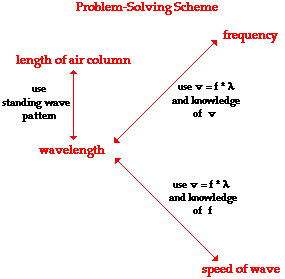To demonstrate the use of the above problem-solving scheme, consider the following example problem and its detailed solution.

### Example Problem #1

The speed of sound waves in air is 340 m/s. Determine the fundamental frequency (1st harmonic) of a closed-end air column that has a length of 67.5 cm.

The solution to the problem begins by first identifying known information, listing the desired quantity, and constructing a diagram of the situation.

 Given: v = 340 m/s L = 67.5 cm = 0.675 m Find: f1 = ?? Diagram: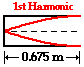The problem statement asks us to determine the frequency (f) value. From the graphic above, the only means of finding the frequency is to use the wave equation (speed=frequency • wavelength) and knowledge of the speed and wavelength. The speed is given, but wavelength is not known. If the wavelength could be found then the frequency could be easily calculated. In this problem (and any problem), knowledge of the length and the harmonic number allows one to determine the wavelength of the wave. For the first harmonic, the wavelength is four times the length. This relationship is derived from the diagram of the standing wave pattern (see table above). The relationship, which works only for the first harmonic of a closed-end air column, is used to calculate the wavelength for this standing wave.

Wavelength = 4 • Length

Wavelength = 4 • 0.675 m

Wavelength = 2.7 m

Now that wavelength is known, it can be combined with the given value of the speed to calculate the frequency of the first harmonic for this closed-end air column. This calculation is shown below.

speed = frequency • wavelength

frequency = speed / wavelength

frequency = (340 m/s) / (2.7 m)

frequency = 126 Hz

Most problems can be solved in a similar manner. It is always wise to take the extra time needed to set the problem up; take the time to write down the given information and the requested information, and to draw a meaningful diagram.

Seldom in physics are two problems identical. The tendency to treat every problem the same way is perhaps one of the quickest paths to failure. It is much better to combine good problem-solving skills (part of which involves the discipline to set the problem up) with a solid grasp of the relationships among variables. Avoid the tendency to memorize approaches to different types of problems.

To further demonstrate the use of the above problem-solving scheme, consider the following example problem and its detailed solution.

### Example Problem #2

Determine the length of an closed-end air column that produces a fundamental frequency (1st harmonic) of 480 Hz. The speed of waves in air is known to be 340 m/s.

The solution to the problem begins by first identifying known information, listing the desired quantity, and constructing a diagram of the situation.

 Given: v = 340 m/s f1 = 480 Hz Find: L = ?? Diagram: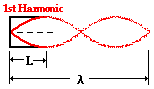The problem statement asks us to determine the length of the air column. From the graphic above, the only means of finding the length of the air column is from knowledge of the wavelength. But the wavelength is not known. However, the frequency and speed are given, so one can use the wave equation (speed = frequency • wavelength) and knowledge of the speed and frequency to determine the wavelength. This calculation is shown below.

speed = frequency • wavelength

wavelength = speed / frequency

wavelength = (340 m/s) / (480 Hz)

wavelength = 0.708 m

Now that the wavelength is found, the length of the air column can be calculated. For the first harmonic, the length is one-fourth the wavelength. This relationship is derived from the diagram of the standing wave pattern (see table above); it may also be evident to you by looking at the standing wave diagram drawn above. This relationship between wavelength and length, which works only for the first harmonic of a closed-end air column, is used to calculate the wavelength for this standing wave.

Length = (1/4) • Wavelength

Length = (1/4) • Wavelength

Length = 0.177 m

If you have successfully followed the logic of the two solutions above, then take a try at the following practice problems. As you proceed, be sure to be mindful of the numerical relationships involved in such problems. And if necessary, refer to the problem solving scheme presented above.

### We Would Like to Suggest ...Why just read about it and when you could be interacting with it? Interact - that's exactly what you do when you use one of The Physics Classroom's Interactives. We would like to suggest that you combine the reading of this page with the use of our Standing Wave Patterns Interactive. You can find it in the Physics Interactives section of our website. The Standing Wave Patterns Interactive provides the learner an environment for exploring the formation of standing waves, standing wave patterns, and mathematical relationships for standing wave patterns.

### Check Your Understanding

1. Titan Tommy and the Test Tubes at a night club this weekend. The lead instrumentalist uses a test tube (closed-end air column) with a 17.2 cm air column. The speed of sound in the test tube is 340 m/sec. Find the frequency of the first harmonic played by this instrument.

2. A closed-end organ pipe is used to produce a mixture of sounds. The third and fifth harmonics in the mixture have frequencies of 1100 Hz and 1833 Hz respectively. What is the frequency of the first harmonic played by the organ pipe?

3. Pipin' Pete is playing at City Park next weekend. One of the closed-end pipes is capable of sounding out a first harmonic of 349.2 Hz. The speed of sound in the pipe is 350 m/sec. Find the length of the air column inside the pipe.

Follow Us